StatLect

# Jensen's inequality

Jensens's inequality is a probabilistic inequality that concerns the expected value of convex and concave transformations of a random variable.## Convex and concave functions

Jensen's inequality applies to convex and concave functions.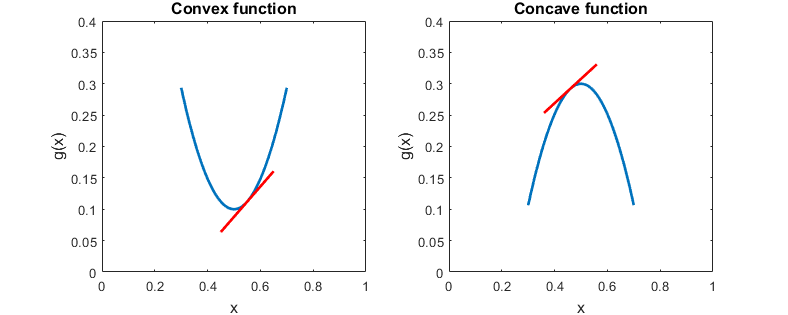The properties of these functions that are relevant for understanding the proof of the inequality are:

• the tangents of a convex function lie entirely below its graph;

• the tangents of a concave function lie entirely above its graph.

Also remember that a differentiable function is:

• (strictly) convex if its second derivative is (strictly) positive;

• (strictly) concave if its second derivative is (strictly) negative.

## Statement

The following is a formal statement of the inequality.

Proposition Letbe an integrable random variable. Letbe a convex function such thatis also integrable. Then, the following inequality, called Jensen's inequality, holds:Proof

A functionis convex if, for any pointthe graph oflies entirely above its tangent at the point: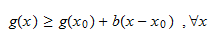whereis the slope of the tangent. Settingand, the inequality becomesBy taking the expected value of both sides of the inequality and using the fact that the expected value operator preserves inequalities, we obtainIf the functionis strictly convex andis not almost surely constant, then we have a strict inequality:Proof

A functionis strictly convex if, for any pointthe graph oflies entirely above its tangent at the point(and strictly so for points different from):whereis the slope of the tangent. Settingand, the inequality becomes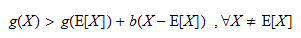and, of course,when. Taking the expected value of both sides of the inequality and using the fact that the expected value operator preserves inequalities, we obtain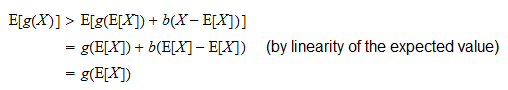where the first inequality is strict because we have assumed thatis not almost surely constant and therefore the eventdoes not have probability.

If the functionis concave, thenProof

Ifis concave, thenis convex and by Jensen's inequality:Multiplying both sides byand using the linearity of the expected value we obtain the result.

If the functionis strictly concave andis not almost surely constant, thenProof

Similar to previous proof.

## Example

Suppose that a strictly positive random variablehas expected valueand it is not constant with probability one.

What can we say about the expected value of, by using Jensen's inequality?

The natural logarithm is a strictly concave function because its second derivativeis strictly negative on its domain of definition.

As a consequence, by Jensen's inequality, we have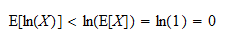Therefore,has a strictly negative expected value.

## Important applications

Jensen's inequality has many applications in statistics. Two important ones are in the proofs of:

## Other inequalities

If you like this page, StatLect has other pages on probabilistic inequalities:

## Solved exercises

Below you can find some exercises with explained solutions.

### Exercise 1

Letbe a random variable having finite mean and variance.

Use Jensen's inequality to find a bound on the expected value of.

Solution

The function we need to study isIt has first derivativeand second derivativeThe second derivative is strictly positive on the domain of definition of the function. Therefore, the function is strictly convex. Furthermore,is not almost surely constant because it has strictly positive variance. Hence, by Jensen's inequality: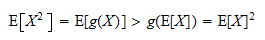Thus, the bound is### Exercise 2

Letbe a positive integrable random variable.

Find a bound on the mean of.

Solution

The function we need to study isIt has first derivativeand second derivativeThe second derivative is negative on the domain of definition of the function. Therefore, the function is concave and Jensen's inequality gives: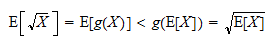Thus, the bound is